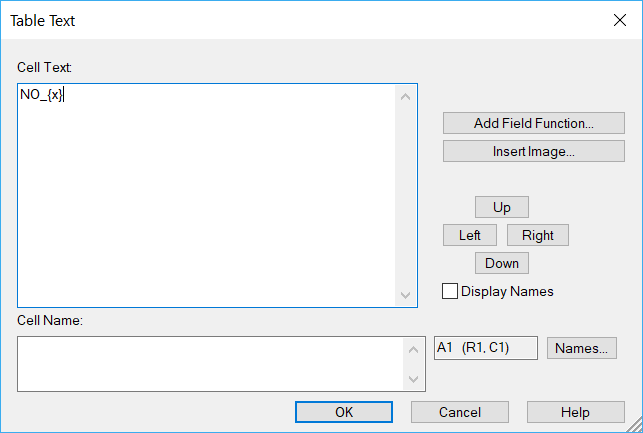Edit Object=>Table¶

In this dialog box, the cell text can be typed in.

The text can have multiple lines. You can use special characters and ANSI character sets. Characters or series of characters can be printed in sub- or superscript. The text can contain lines, arrows and symbols.Text Field

Edit text in this box.

Using this button a field function can be selected from a dialog box.

Name

The cell name can contain a placeholder. A placeholder is text that begins with a dollar sign, followed by the text, followed by another dollar sign, e.g. \$Operator\$.

The placeholders can be edited using a special dialog box or their text can be loaded from a data file using the data browser or using the command Load Placeholder Text form file (Right click outside the page to open the page context menu.)

To load the data from a data file the text within the \$ symbols must agree with text in the global attributes of the selected NC file. The placeholder text is case sensitive. Values will be transformed into strings. If the NC attribute contains a vector, the elements will separated by a comma followed by a blank.

To access attributes of a specific variable the variable name must be separated from the attribute name with a period. Example: \$Torque.long_name\$.

The placeholder text can be loaded from a data file using the data browser. See The Data Browser. Select the type Text: Load Placeholder Text.

Display Names
If this option is selected the cell names will be temporarily displayed in the table cells until the dialog box is closed.
Left, Right, Top, Bottom
These buttons are used to navigate to other cells without leaving the dialog box.

Comment

In the text of an object you can use special characters and the ANSI character sets. Characters or series of characters can be printed in sub- or superscript. Text can contain lines, arrows and symbols. See Text Objects.

Field functions are especially coded strings in text fields. They can be used to update information in documents automatically. They can be used with table or text drawing objects.

Example: The file name is displayed as a text box in a UniPlot document page. If the document is saved under a new name the field is updated and displays the new name.

Solution: Create a text box and type the following text: @f{documentname}. Instead of the string @f{documentname} the file name is displayed, e.g. c:\test.ipw.

Simple Table Calculations

The cell name can contain a formula. The formula always starts with an equal sign (=), which can be followed by numbers, math operators (+ - * / ^) and UniScript functions. The formula must be added to the name element of a table cell. The result of a formula must be a single real or string value.

A formula can only reference cells in the same table. Cells in other tables cannot be accessed.

Here are some formula examples:

Example Description
=A1+A2+A3 Adds the values in cells A1, A2 and A3.
=sum(A1:A12) Calculates the sum of the values in A1 through A12
=IF(A1 > 10, A2, B2) Uses the IF function to test, if the A1 cell is greater than 10. If true, A2 is used otherwise B2. Valid comparision operators: >, <, >=, <=, !=, == Boolean operators can be used: && (AND) || (OR) ! (NOT)
=(A1+A2+A3)/3 Calculates the avarage of A1, A2 and A3.
=mean(A1:A12) Calculates the avarage of A1 to A12. Colons (:) are used to separate the reference to the first and last cell in the range
=sprintf(“%.2lf kg”, mean(A1:A12)) Calculates the avarage of A1 to A12 and formats the result. 2 decimals places plus the unit kg.
=std(A1:A12) Calculates the standard deviation of A1 through A12.
=FormatCondition(F4 > 0.5, "#00FF7F",  "#FF7F7F", "Passed", "Failed") Highlight a cell. If the cell value of F4 is greater than 0.5 the cell color is set to green (“#00FF7F”) and the value Passed is displayed. Otherwise the cell is display in red with the text Failed.

The cell reference A1 refers to the value of the table cell in column 1 (A) and row 1. A20:A30 is a range of cells in column 1 (A) and rows 20 through 30.

If a cell does not contain a valid number, it will be converted to the value 0. The formula can contain parentheses.

FormatCondition(rsCompare, color_true, color_false, pValue_true, pValue_false)¶

Sets the cell color.

A color can be spefied by its name (“red”), as an RGB value [255,0,0] or using its hex-code #000000 (“#FF0000”).

The rsCompare parameter contains the condion, e.g. that a cell value is greater than a constant value, e.g F4 > 0.5. Other compare operators: <, == , !=, >=, <=. Logical operators can be used: AND (&&), OR (||), e.g. F4 > 0.5 || F5 > 1.5

If the function is called with 3 parameters, the cell text will not be changed. If the function is called with 4 parameters, pValue_true will be displayed. If the function is called with 5 parameters, pValue_true will be displayed, if the condition is TRUE (1) and otherwise the pValue_false will be displayed. angezeigt.

IF(rsCompare, value_true, value_false)¶

If rsCompare is TRUE (1), e.g. F4 > 0.5 the function returns value_true and otherwise value_false. The value can be a string or number.

User defined table functions¶

New functions can be added. See FormatCondition in the file <uniplot>scriptdrawingrs_table_evaluate.ic an an example. The function must return a scalar string or number. Teh return value will be displayed in the cell.

id-415001